# Stacked Regressions to predict House Prices¶

## Serigne¶

July 2017

If you use parts of this notebook in your scripts/notebooks, giving some kind of credit would be very much appreciated :) You can for instance link back to this notebook. Thanks!

This competition is very important to me as it helped me to begin my journey on Kaggle few months ago. I've read some great notebooks here. To name a few:

1. Comprehensive data exploration with Python by Pedro Marcelino : Great and very motivational data analysis

2. A study on Regression applied to the Ames dataset by Julien Cohen-Solal : Thorough features engeneering and deep dive into linear regression analysis but really easy to follow for beginners.

3. Regularized Linear Models by Alexandru Papiu : Great Starter kernel on modelling and Cross-validation

I can't recommend enough every beginner to go carefully through these kernels (and of course through many others great kernels) and get their first insights in data science and kaggle competitions.

After that (and some basic pratices) you should be more confident to go through this great script by Human Analog who did an impressive work on features engeneering.

As the dataset is particularly handy, I decided few days ago to get back in this competition and apply things I learnt so far, especially stacking models. For that purpose, we build two stacking classes ( the simplest approach and a less simple one).

As these classes are written for general purpose, you can easily adapt them and/or extend them for your regression problems. The overall approach is hopefully concise and easy to follow..

The features engeneering is rather parsimonious (at least compared to some others great scripts) . It is pretty much :

• Imputing missing values by proceeding sequentially through the data

• Transforming some numerical variables that seem really categorical

• Label Encoding some categorical variables that may contain information in their ordering set

• Box Cox Transformation of skewed features (instead of log-transformation) : This gave me a slightly better result both on leaderboard and cross-validation.

• Getting dummy variables for categorical features.

Then we choose many base models (mostly sklearn based models + sklearn API of DMLC's XGBoost and Microsoft's LightGBM), cross-validate them on the data before stacking/ensembling them. The key here is to make the (linear) models robust to outliers. This improved the result both on LB and cross-validation.

To my surprise, this does well on LB ( 0.11420 and top 4% the last time I tested it : July 2, 2017 )

Hope that at the end of this notebook, stacking will be clear for those, like myself, who found the concept not so easy to grasp

In :
#import some necessary librairies

import numpy as np # linear algebra
import pandas as pd # data processing, CSV file I/O (e.g. pd.read_csv)
%matplotlib inline
import matplotlib.pyplot as plt  # Matlab-style plotting
import seaborn as sns
color = sns.color_palette()
sns.set_style('darkgrid')
import warnings
def ignore_warn(*args, **kwargs):
pass
warnings.warn = ignore_warn #ignore annoying warning (from sklearn and seaborn)

from scipy import stats
from scipy.stats import norm, skew #for some statistics

pd.set_option('display.float_format', lambda x: '{:.3f}'.format(x)) #Limiting floats output to 3 decimal points

from subprocess import check_output
print(check_output(["ls", "../input"]).decode("utf8")) #check the files available in the directory

sample_submission.csv
test.csv
train.csv


In :
#Now let's import and put the train and test datasets in  pandas dataframe


In :
##display the first five rows of the train dataset.

Out:
Id MSSubClass MSZoning LotFrontage LotArea Street Alley LotShape LandContour Utilities ... PoolArea PoolQC Fence MiscFeature MiscVal MoSold YrSold SaleType SaleCondition SalePrice
0 1 60 RL 65.000 8450 Pave NaN Reg Lvl AllPub ... 0 NaN NaN NaN 0 2 2008 WD Normal 208500
1 2 20 RL 80.000 9600 Pave NaN Reg Lvl AllPub ... 0 NaN NaN NaN 0 5 2007 WD Normal 181500
2 3 60 RL 68.000 11250 Pave NaN IR1 Lvl AllPub ... 0 NaN NaN NaN 0 9 2008 WD Normal 223500
3 4 70 RL 60.000 9550 Pave NaN IR1 Lvl AllPub ... 0 NaN NaN NaN 0 2 2006 WD Abnorml 140000
4 5 60 RL 84.000 14260 Pave NaN IR1 Lvl AllPub ... 0 NaN NaN NaN 0 12 2008 WD Normal 250000

5 rows × 81 columns

In :
##display the first five rows of the test dataset.

Out:
Id MSSubClass MSZoning LotFrontage LotArea Street Alley LotShape LandContour Utilities ... ScreenPorch PoolArea PoolQC Fence MiscFeature MiscVal MoSold YrSold SaleType SaleCondition
0 1461 20 RH 80.000 11622 Pave NaN Reg Lvl AllPub ... 120 0 NaN MnPrv NaN 0 6 2010 WD Normal
1 1462 20 RL 81.000 14267 Pave NaN IR1 Lvl AllPub ... 0 0 NaN NaN Gar2 12500 6 2010 WD Normal
2 1463 60 RL 74.000 13830 Pave NaN IR1 Lvl AllPub ... 0 0 NaN MnPrv NaN 0 3 2010 WD Normal
3 1464 60 RL 78.000 9978 Pave NaN IR1 Lvl AllPub ... 0 0 NaN NaN NaN 0 6 2010 WD Normal
4 1465 120 RL 43.000 5005 Pave NaN IR1 HLS AllPub ... 144 0 NaN NaN NaN 0 1 2010 WD Normal

5 rows × 80 columns

In :
#check the numbers of samples and features
print("The train data size before dropping Id feature is : {} ".format(train.shape))
print("The test data size before dropping Id feature is : {} ".format(test.shape))

#Save the 'Id' column
train_ID = train['Id']
test_ID = test['Id']

#Now drop the  'Id' colum since it's unnecessary for  the prediction process.
train.drop("Id", axis = 1, inplace = True)
test.drop("Id", axis = 1, inplace = True)

#check again the data size after dropping the 'Id' variable
print("\nThe train data size after dropping Id feature is : {} ".format(train.shape))
print("The test data size after dropping Id feature is : {} ".format(test.shape))

The train data size before dropping Id feature is : (1460, 81)
The test data size before dropping Id feature is : (1459, 80)

The train data size after dropping Id feature is : (1460, 80)
The test data size after dropping Id feature is : (1459, 79)


# Data Processing¶

## Outliers¶

Documentation for the Ames Housing Data indicates that there are outliers present in the training data

Let's explore these outliers

In :
fig, ax = plt.subplots()
ax.scatter(x = train['GrLivArea'], y = train['SalePrice'])
plt.ylabel('SalePrice', fontsize=13)
plt.xlabel('GrLivArea', fontsize=13)
plt.show()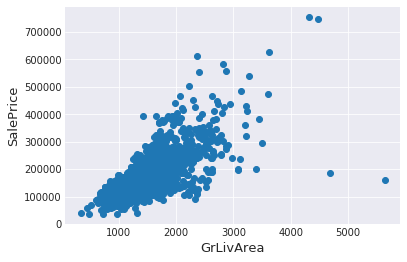We can see at the bottom right two with extremely large GrLivArea that are of a low price. These values are huge oultliers. Therefore, we can safely delete them.

In :
#Deleting outliers
train = train.drop(train[(train['GrLivArea']>4000) & (train['SalePrice']<300000)].index)

#Check the graphic again
fig, ax = plt.subplots()
ax.scatter(train['GrLivArea'], train['SalePrice'])
plt.ylabel('SalePrice', fontsize=13)
plt.xlabel('GrLivArea', fontsize=13)
plt.show()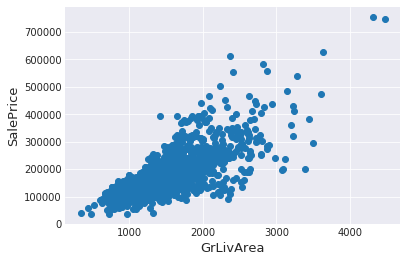### Note :¶

Outliers removal is note always safe. We decided to delete these two as they are very huge and really bad ( extremely large areas for very low prices).

There are probably others outliers in the training data. However, removing all them may affect badly our models if ever there were also outliers in the test data. That's why , instead of removing them all, we will just manage to make some of our models robust on them. You can refer to the modelling part of this notebook for that.

## Target Variable¶

SalePrice is the variable we need to predict. So let's do some analysis on this variable first.

In :
sns.distplot(train['SalePrice'] , fit=norm);

# Get the fitted parameters used by the function
(mu, sigma) = norm.fit(train['SalePrice'])
print( '\n mu = {:.2f} and sigma = {:.2f}\n'.format(mu, sigma))

#Now plot the distribution
plt.legend(['Normal dist. ($\mu=$ {:.2f} and $\sigma=$ {:.2f} )'.format(mu, sigma)],
loc='best')
plt.ylabel('Frequency')
plt.title('SalePrice distribution')

#Get also the QQ-plot
fig = plt.figure()
res = stats.probplot(train['SalePrice'], plot=plt)
plt.show()

 mu = 180932.92 and sigma = 79467.79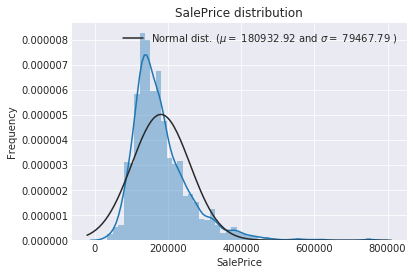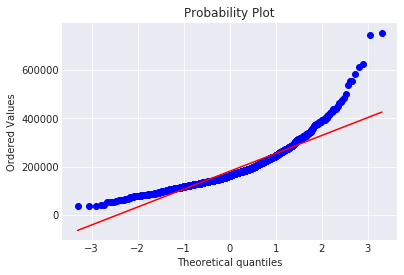The target variable is right skewed. As (linear) models love normally distributed data , we need to transform this variable and make it more normally distributed.

Log-transformation of the target variable

In :
#We use the numpy fuction log1p which  applies log(1+x) to all elements of the column
train["SalePrice"] = np.log1p(train["SalePrice"])

#Check the new distribution
sns.distplot(train['SalePrice'] , fit=norm);

# Get the fitted parameters used by the function
(mu, sigma) = norm.fit(train['SalePrice'])
print( '\n mu = {:.2f} and sigma = {:.2f}\n'.format(mu, sigma))

#Now plot the distribution
plt.legend(['Normal dist. ($\mu=$ {:.2f} and $\sigma=$ {:.2f} )'.format(mu, sigma)],
loc='best')
plt.ylabel('Frequency')
plt.title('SalePrice distribution')

#Get also the QQ-plot
fig = plt.figure()
res = stats.probplot(train['SalePrice'], plot=plt)
plt.show()

 mu = 12.02 and sigma = 0.40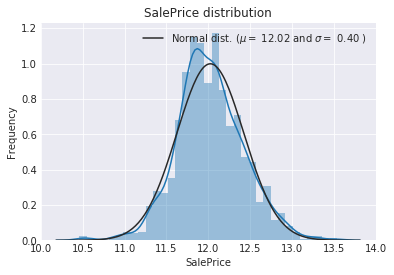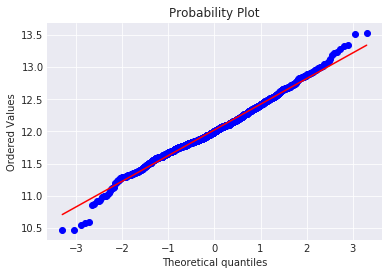The skew seems now corrected and the data appears more normally distributed.

## Features engineering¶

let's first concatenate the train and test data in the same dataframe

In :
ntrain = train.shape
ntest = test.shape
y_train = train.SalePrice.values
all_data = pd.concat((train, test)).reset_index(drop=True)
all_data.drop(['SalePrice'], axis=1, inplace=True)
print("all_data size is : {}".format(all_data.shape))

all_data size is : (2917, 79)


### Missing Data¶

In :
all_data_na = (all_data.isnull().sum() / len(all_data)) * 100
all_data_na = all_data_na.drop(all_data_na[all_data_na == 0].index).sort_values(ascending=False)[:30]
missing_data = pd.DataFrame({'Missing Ratio' :all_data_na})

Out:
Missing Ratio
PoolQC 99.691
MiscFeature 96.400
Alley 93.212
Fence 80.425
FireplaceQu 48.680
LotFrontage 16.661
GarageQual 5.451
GarageCond 5.451
GarageFinish 5.451
GarageYrBlt 5.451
GarageType 5.382
BsmtExposure 2.811
BsmtCond 2.811
BsmtQual 2.777
BsmtFinType2 2.743
BsmtFinType1 2.708
MasVnrType 0.823
MasVnrArea 0.788
MSZoning 0.137
BsmtFullBath 0.069
In :
f, ax = plt.subplots(figsize=(15, 12))
plt.xticks(rotation='90')
sns.barplot(x=all_data_na.index, y=all_data_na)
plt.xlabel('Features', fontsize=15)
plt.ylabel('Percent of missing values', fontsize=15)
plt.title('Percent missing data by feature', fontsize=15)

Out:
Text(0.5,1,'Percent missing data by feature')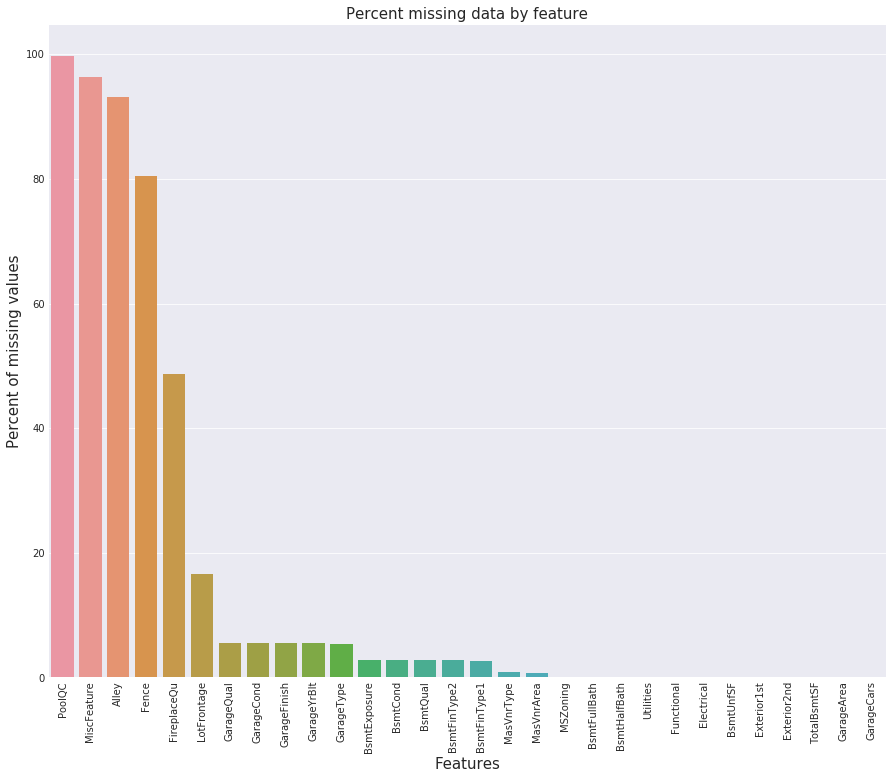Data Correlation

In :
#Correlation map to see how features are correlated with SalePrice
corrmat = train.corr()
plt.subplots(figsize=(12,9))
sns.heatmap(corrmat, vmax=0.9, square=True)

Out:
<matplotlib.axes._subplots.AxesSubplot at 0x7efd7b454898>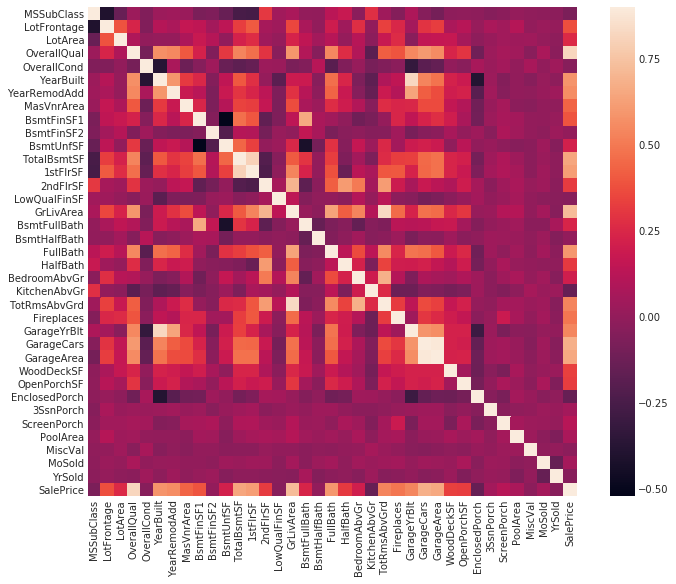### Imputing missing values¶

We impute them by proceeding sequentially through features with missing values

• PoolQC : data description says NA means "No Pool". That make sense, given the huge ratio of missing value (+99%) and majority of houses have no Pool at all in general.
In :
all_data["PoolQC"] = all_data["PoolQC"].fillna("None")

• MiscFeature : data description says NA means "no misc feature"
In :
all_data["MiscFeature"] = all_data["MiscFeature"].fillna("None")

• Alley : data description says NA means "no alley access"
In :
all_data["Alley"] = all_data["Alley"].fillna("None")

• Fence : data description says NA means "no fence"
In :
all_data["Fence"] = all_data["Fence"].fillna("None")

• FireplaceQu : data description says NA means "no fireplace"
In :
all_data["FireplaceQu"] = all_data["FireplaceQu"].fillna("None")

• LotFrontage : Since the area of each street connected to the house property most likely have a similar area to other houses in its neighborhood , we can fill in missing values by the median LotFrontage of the neighborhood.
In :
#Group by neighborhood and fill in missing value by the median LotFrontage of all the neighborhood
all_data["LotFrontage"] = all_data.groupby("Neighborhood")["LotFrontage"].transform(
lambda x: x.fillna(x.median()))

• GarageType, GarageFinish, GarageQual and GarageCond : Replacing missing data with None
In :
for col in ('GarageType', 'GarageFinish', 'GarageQual', 'GarageCond'):
all_data[col] = all_data[col].fillna('None')

• GarageYrBlt, GarageArea and GarageCars : Replacing missing data with 0 (Since No garage = no cars in such garage.)
In :
for col in ('GarageYrBlt', 'GarageArea', 'GarageCars'):
all_data[col] = all_data[col].fillna(0)

• BsmtFinSF1, BsmtFinSF2, BsmtUnfSF, TotalBsmtSF, BsmtFullBath and BsmtHalfBath : missing values are likely zero for having no basement
In :
for col in ('BsmtFinSF1', 'BsmtFinSF2', 'BsmtUnfSF','TotalBsmtSF', 'BsmtFullBath', 'BsmtHalfBath'):
all_data[col] = all_data[col].fillna(0)

• BsmtQual, BsmtCond, BsmtExposure, BsmtFinType1 and BsmtFinType2 : For all these categorical basement-related features, NaN means that there is no basement.
In :
for col in ('BsmtQual', 'BsmtCond', 'BsmtExposure', 'BsmtFinType1', 'BsmtFinType2'):
all_data[col] = all_data[col].fillna('None')

• MasVnrArea and MasVnrType : NA most likely means no masonry veneer for these houses. We can fill 0 for the area and None for the type.
In :
all_data["MasVnrType"] = all_data["MasVnrType"].fillna("None")
all_data["MasVnrArea"] = all_data["MasVnrArea"].fillna(0)

• MSZoning (The general zoning classification) : 'RL' is by far the most common value. So we can fill in missing values with 'RL'
In :
all_data['MSZoning'] = all_data['MSZoning'].fillna(all_data['MSZoning'].mode())

• Utilities : For this categorical feature all records are "AllPub", except for one "NoSeWa" and 2 NA . Since the house with 'NoSewa' is in the training set, this feature won't help in predictive modelling. We can then safely remove it.
In :
all_data = all_data.drop(['Utilities'], axis=1)

• Functional : data description says NA means typical
In :
all_data["Functional"] = all_data["Functional"].fillna("Typ")

• Electrical : It has one NA value. Since this feature has mostly 'SBrkr', we can set that for the missing value.
In :
all_data['Electrical'] = all_data['Electrical'].fillna(all_data['Electrical'].mode())

• KitchenQual: Only one NA value, and same as Electrical, we set 'TA' (which is the most frequent) for the missing value in KitchenQual.
In :
all_data['KitchenQual'] = all_data['KitchenQual'].fillna(all_data['KitchenQual'].mode())

• Exterior1st and Exterior2nd : Again Both Exterior 1 & 2 have only one missing value. We will just substitute in the most common string
In :
all_data['Exterior1st'] = all_data['Exterior1st'].fillna(all_data['Exterior1st'].mode())
all_data['Exterior2nd'] = all_data['Exterior2nd'].fillna(all_data['Exterior2nd'].mode())

• SaleType : Fill in again with most frequent which is "WD"
In :
all_data['SaleType'] = all_data['SaleType'].fillna(all_data['SaleType'].mode())

• MSSubClass : Na most likely means No building class. We can replace missing values with None
In :
all_data['MSSubClass'] = all_data['MSSubClass'].fillna("None")


Is there any remaining missing value ?

In :
#Check remaining missing values if any
all_data_na = (all_data.isnull().sum() / len(all_data)) * 100
all_data_na = all_data_na.drop(all_data_na[all_data_na == 0].index).sort_values(ascending=False)
missing_data = pd.DataFrame({'Missing Ratio' :all_data_na})

Out:
Missing Ratio

It remains no missing value.

### More features engeneering¶

Transforming some numerical variables that are really categorical

In :
#MSSubClass=The building class
all_data['MSSubClass'] = all_data['MSSubClass'].apply(str)

#Changing OverallCond into a categorical variable
all_data['OverallCond'] = all_data['OverallCond'].astype(str)

#Year and month sold are transformed into categorical features.
all_data['YrSold'] = all_data['YrSold'].astype(str)
all_data['MoSold'] = all_data['MoSold'].astype(str)


Label Encoding some categorical variables that may contain information in their ordering set

In :
from sklearn.preprocessing import LabelEncoder
cols = ('FireplaceQu', 'BsmtQual', 'BsmtCond', 'GarageQual', 'GarageCond',
'ExterQual', 'ExterCond','HeatingQC', 'PoolQC', 'KitchenQual', 'BsmtFinType1',
'BsmtFinType2', 'Functional', 'Fence', 'BsmtExposure', 'GarageFinish', 'LandSlope',
'LotShape', 'PavedDrive', 'Street', 'Alley', 'CentralAir', 'MSSubClass', 'OverallCond',
'YrSold', 'MoSold')
# process columns, apply LabelEncoder to categorical features
for c in cols:
lbl = LabelEncoder()
lbl.fit(list(all_data[c].values))
all_data[c] = lbl.transform(list(all_data[c].values))

# shape
print('Shape all_data: {}'.format(all_data.shape))

Shape all_data: (2917, 78)


Adding one more important feature

Since area related features are very important to determine house prices, we add one more feature which is the total area of basement, first and second floor areas of each house

In :
# Adding total sqfootage feature
all_data['TotalSF'] = all_data['TotalBsmtSF'] + all_data['1stFlrSF'] + all_data['2ndFlrSF']


Skewed features

In :
numeric_feats = all_data.dtypes[all_data.dtypes != "object"].index

# Check the skew of all numerical features
skewed_feats = all_data[numeric_feats].apply(lambda x: skew(x.dropna())).sort_values(ascending=False)
print("\nSkew in numerical features: \n")
skewness = pd.DataFrame({'Skew' :skewed_feats})

Skew in numerical features:


Out:
Skew
MiscVal 21.940
PoolArea 17.689
LotArea 13.109
LowQualFinSF 12.085
3SsnPorch 11.372
LandSlope 4.973
KitchenAbvGr 4.301
BsmtFinSF2 4.145
EnclosedPorch 4.002
ScreenPorch 3.945

Box Cox Transformation of (highly) skewed features

We use the scipy function boxcox1p which computes the Box-Cox transformation of $1 + x$.

Note that setting $\lambda = 0$ is equivalent to log1p used above for the target variable.

See this page for more details on Box Cox Transformation as well as the scipy function's page

In :
skewness = skewness[abs(skewness) > 0.75]
print("There are {} skewed numerical features to Box Cox transform".format(skewness.shape))

from scipy.special import boxcox1p
skewed_features = skewness.index
lam = 0.15
for feat in skewed_features:
#all_data[feat] += 1
all_data[feat] = boxcox1p(all_data[feat], lam)

#all_data[skewed_features] = np.log1p(all_data[skewed_features])

There are 59 skewed numerical features to Box Cox transform


Getting dummy categorical features

In :
all_data = pd.get_dummies(all_data)
print(all_data.shape)

(2917, 220)


Getting the new train and test sets.

In :
train = all_data[:ntrain]
test = all_data[ntrain:]


# Modelling¶

Import librairies

In :
from sklearn.linear_model import ElasticNet, Lasso,  BayesianRidge, LassoLarsIC
from sklearn.ensemble import RandomForestRegressor,  GradientBoostingRegressor
from sklearn.kernel_ridge import KernelRidge
from sklearn.pipeline import make_pipeline
from sklearn.preprocessing import RobustScaler
from sklearn.base import BaseEstimator, TransformerMixin, RegressorMixin, clone
from sklearn.model_selection import KFold, cross_val_score, train_test_split
from sklearn.metrics import mean_squared_error
import xgboost as xgb
import lightgbm as lgb


Define a cross validation strategy

We use the cross_val_score function of Sklearn. However this function has not a shuffle attribut, we add then one line of code, in order to shuffle the dataset prior to cross-validation

In :
#Validation function
n_folds = 5

def rmsle_cv(model):
kf = KFold(n_folds, shuffle=True, random_state=42).get_n_splits(train.values)
rmse= np.sqrt(-cross_val_score(model, train.values, y_train, scoring="neg_mean_squared_error", cv = kf))
return(rmse)


## Base models¶

• LASSO Regression :

This model may be very sensitive to outliers. So we need to made it more robust on them. For that we use the sklearn's Robustscaler() method on pipeline

In :
lasso = make_pipeline(RobustScaler(), Lasso(alpha =0.0005, random_state=1))

• Elastic Net Regression :

again made robust to outliers

In :
ENet = make_pipeline(RobustScaler(), ElasticNet(alpha=0.0005, l1_ratio=.9, random_state=3))

• Kernel Ridge Regression :
In :
KRR = KernelRidge(alpha=0.6, kernel='polynomial', degree=2, coef0=2.5)

• Gradient Boosting Regression :

With huber loss that makes it robust to outliers

In :
GBoost = GradientBoostingRegressor(n_estimators=3000, learning_rate=0.05,
max_depth=4, max_features='sqrt',
min_samples_leaf=15, min_samples_split=10,
loss='huber', random_state =5)

• XGBoost :
In :
model_xgb = xgb.XGBRegressor(colsample_bytree=0.4603, gamma=0.0468,
learning_rate=0.05, max_depth=3,
min_child_weight=1.7817, n_estimators=2200,
reg_alpha=0.4640, reg_lambda=0.8571,
subsample=0.5213, silent=1,
random_state =7, nthread = -1)

• LightGBM :
In :
model_lgb = lgb.LGBMRegressor(objective='regression',num_leaves=5,
learning_rate=0.05, n_estimators=720,
max_bin = 55, bagging_fraction = 0.8,
bagging_freq = 5, feature_fraction = 0.2319,
feature_fraction_seed=9, bagging_seed=9,
min_data_in_leaf =6, min_sum_hessian_in_leaf = 11)


### Base models scores¶

Let's see how these base models perform on the data by evaluating the cross-validation rmsle error

In :
score = rmsle_cv(lasso)
print("\nLasso score: {:.4f} ({:.4f})\n".format(score.mean(), score.std()))

Lasso score: 0.1115 (0.0074)


In :
score = rmsle_cv(ENet)
print("ElasticNet score: {:.4f} ({:.4f})\n".format(score.mean(), score.std()))

ElasticNet score: 0.1116 (0.0074)


In :
score = rmsle_cv(KRR)
print("Kernel Ridge score: {:.4f} ({:.4f})\n".format(score.mean(), score.std()))

Kernel Ridge score: 0.1153 (0.0075)


In :
score = rmsle_cv(GBoost)
print("Gradient Boosting score: {:.4f} ({:.4f})\n".format(score.mean(), score.std()))

Gradient Boosting score: 0.1177 (0.0080)


In :
score = rmsle_cv(model_xgb)
print("Xgboost score: {:.4f} ({:.4f})\n".format(score.mean(), score.std()))

Xgboost score: 0.1161 (0.0079)


In :
score = rmsle_cv(model_lgb)
print("LGBM score: {:.4f} ({:.4f})\n" .format(score.mean(), score.std()))

LGBM score: 0.1157 (0.0067)



## Stacking models¶

### Simplest Stacking approach : Averaging base models¶

We begin with this simple approach of averaging base models. We build a new class to extend scikit-learn with our model and also to laverage encapsulation and code reuse (inheritance)

Averaged base models class

In :
class AveragingModels(BaseEstimator, RegressorMixin, TransformerMixin):
def __init__(self, models):
self.models = models

# we define clones of the original models to fit the data in
def fit(self, X, y):
self.models_ = [clone(x) for x in self.models]

# Train cloned base models
for model in self.models_:
model.fit(X, y)

return self

#Now we do the predictions for cloned models and average them
def predict(self, X):
predictions = np.column_stack([
model.predict(X) for model in self.models_
])
return np.mean(predictions, axis=1)


Averaged base models score

We just average four models here ENet, GBoost, KRR and lasso. Of course we could easily add more models in the mix.

In :
averaged_models = AveragingModels(models = (ENet, GBoost, KRR, lasso))

score = rmsle_cv(averaged_models)
print(" Averaged base models score: {:.4f} ({:.4f})\n".format(score.mean(), score.std()))

 Averaged base models score: 0.1091 (0.0075)



Wow ! It seems even the simplest stacking approach really improve the score . This encourages us to go further and explore a less simple stacking approch.

### Less simple Stacking : Adding a Meta-model¶

In this approach, we add a meta-model on averaged base models and use the out-of-folds predictions of these base models to train our meta-model.

The procedure, for the training part, may be described as follows:

1. Split the total training set into two disjoint sets (here train and .holdout )

2. Train several base models on the first part (train)

3. Test these base models on the second part (holdout)

4. Use the predictions from 3) (called out-of-folds predictions) as the inputs, and the correct responses (target variable) as the outputs to train a higher level learner called meta-model.

The first three steps are done iteratively . If we take for example a 5-fold stacking , we first split the training data into 5 folds. Then we will do 5 iterations. In each iteration, we train every base model on 4 folds and predict on the remaining fold (holdout fold).

So, we will be sure, after 5 iterations , that the entire data is used to get out-of-folds predictions that we will then use as new feature to train our meta-model in the step 4.

For the prediction part , We average the predictions of all base models on the test data and used them as meta-features on which, the final prediction is done with the meta-model.(Image taken from Faron)Gif taken from KazAnova's interview

On this gif, the base models are algorithms 0, 1, 2 and the meta-model is algorithm 3. The entire training dataset is A+B (target variable y known) that we can split into train part (A) and holdout part (B). And the test dataset is C.

B1 (which is the prediction from the holdout part) is the new feature used to train the meta-model 3 and C1 (which is the prediction from the test dataset) is the meta-feature on which the final prediction is done.

Stacking averaged Models Class

In :
class StackingAveragedModels(BaseEstimator, RegressorMixin, TransformerMixin):
def __init__(self, base_models, meta_model, n_folds=5):
self.base_models = base_models
self.meta_model = meta_model
self.n_folds = n_folds

# We again fit the data on clones of the original models
def fit(self, X, y):
self.base_models_ = [list() for x in self.base_models]
self.meta_model_ = clone(self.meta_model)
kfold = KFold(n_splits=self.n_folds, shuffle=True, random_state=156)

# Train cloned base models then create out-of-fold predictions
# that are needed to train the cloned meta-model
out_of_fold_predictions = np.zeros((X.shape, len(self.base_models)))
for i, model in enumerate(self.base_models):
for train_index, holdout_index in kfold.split(X, y):
instance = clone(model)
self.base_models_[i].append(instance)
instance.fit(X[train_index], y[train_index])
y_pred = instance.predict(X[holdout_index])
out_of_fold_predictions[holdout_index, i] = y_pred

# Now train the cloned  meta-model using the out-of-fold predictions as new feature
self.meta_model_.fit(out_of_fold_predictions, y)
return self

#Do the predictions of all base models on the test data and use the averaged predictions as
#meta-features for the final prediction which is done by the meta-model
def predict(self, X):
meta_features = np.column_stack([
np.column_stack([model.predict(X) for model in base_models]).mean(axis=1)
for base_models in self.base_models_ ])
return self.meta_model_.predict(meta_features)


Stacking Averaged models Score

To make the two approaches comparable (by using the same number of models) , we just average Enet KRR and Gboost, then we add lasso as meta-model.

In :
stacked_averaged_models = StackingAveragedModels(base_models = (ENet, GBoost, KRR),
meta_model = lasso)

score = rmsle_cv(stacked_averaged_models)
print("Stacking Averaged models score: {:.4f} ({:.4f})".format(score.mean(), score.std()))

Stacking Averaged models score: 0.1085 (0.0074)


We get again a better score by adding a meta learner

## Ensembling StackedRegressor, XGBoost and LightGBM¶

We add XGBoost and LightGBM to the StackedRegressor defined previously.

We first define a rmsle evaluation function

In :
def rmsle(y, y_pred):
return np.sqrt(mean_squared_error(y, y_pred))


### Final Training and Prediction¶

StackedRegressor:

In :
stacked_averaged_models.fit(train.values, y_train)
stacked_train_pred = stacked_averaged_models.predict(train.values)
stacked_pred = np.expm1(stacked_averaged_models.predict(test.values))
print(rmsle(y_train, stacked_train_pred))

0.0781571937916


XGBoost:

In :
model_xgb.fit(train, y_train)
xgb_train_pred = model_xgb.predict(train)
xgb_pred = np.expm1(model_xgb.predict(test))
print(rmsle(y_train, xgb_train_pred))

0.0785165142425


LightGBM:

In :
model_lgb.fit(train, y_train)
lgb_train_pred = model_lgb.predict(train)
lgb_pred = np.expm1(model_lgb.predict(test.values))
print(rmsle(y_train, lgb_train_pred))

0.0716757468834

In :
'''RMSE on the entire Train data when averaging'''

print('RMSLE score on train data:')
print(rmsle(y_train,stacked_train_pred*0.70 +
xgb_train_pred*0.15 + lgb_train_pred*0.15 ))

RMSLE score on train data:
0.0752190464543


Ensemble prediction:

In :
ensemble = stacked_pred*0.70 + xgb_pred*0.15 + lgb_pred*0.15


Submission

In :
sub = pd.DataFrame()
sub['Id'] = test_ID
sub['SalePrice'] = ensemble
sub.to_csv('submission.csv',index=False)


If you found this notebook helpful or you just liked it , some upvotes would be very much appreciated - That will keep me motivated to update it on a regular basis :-)